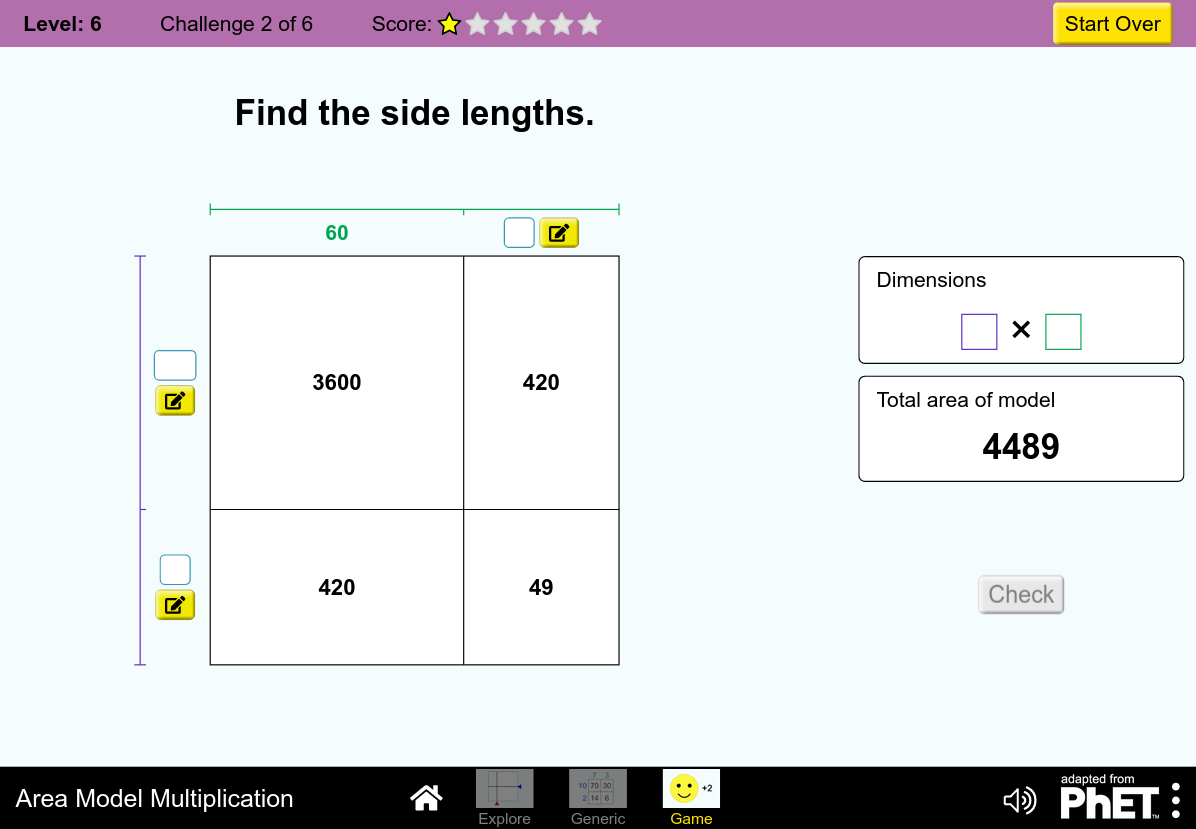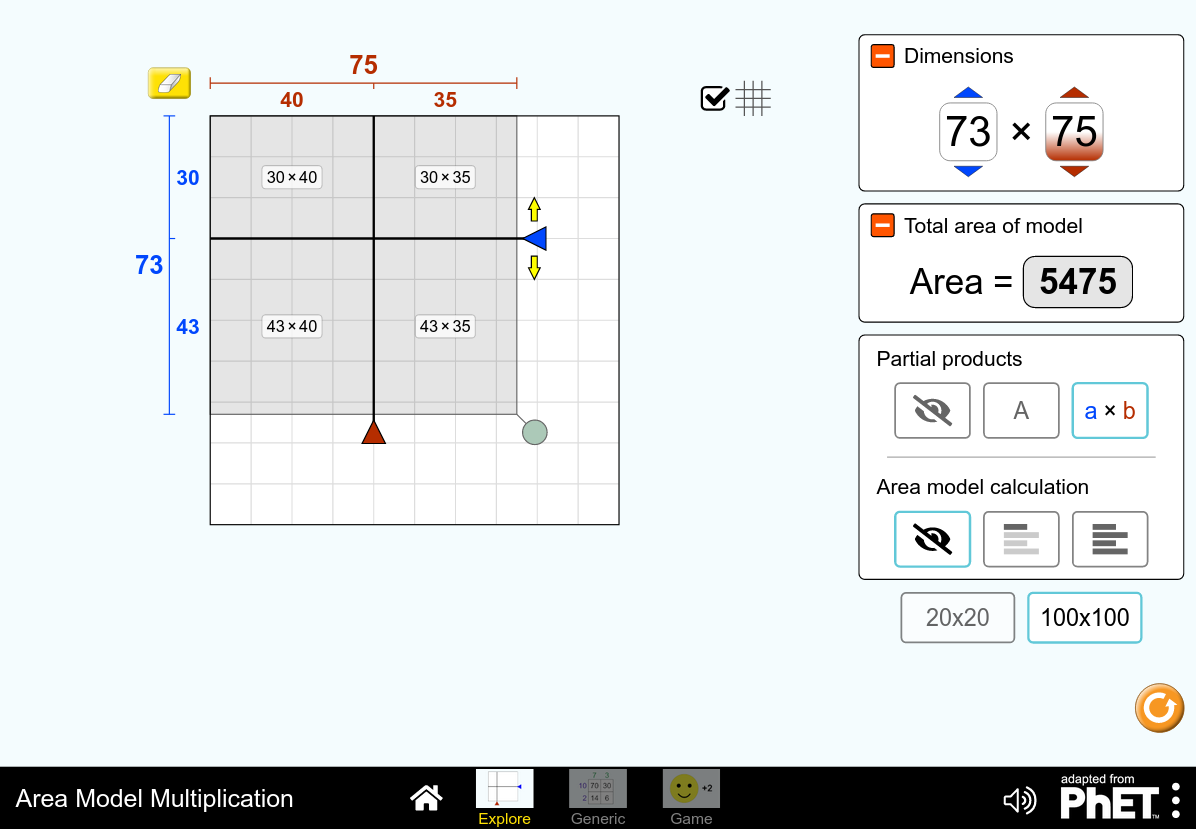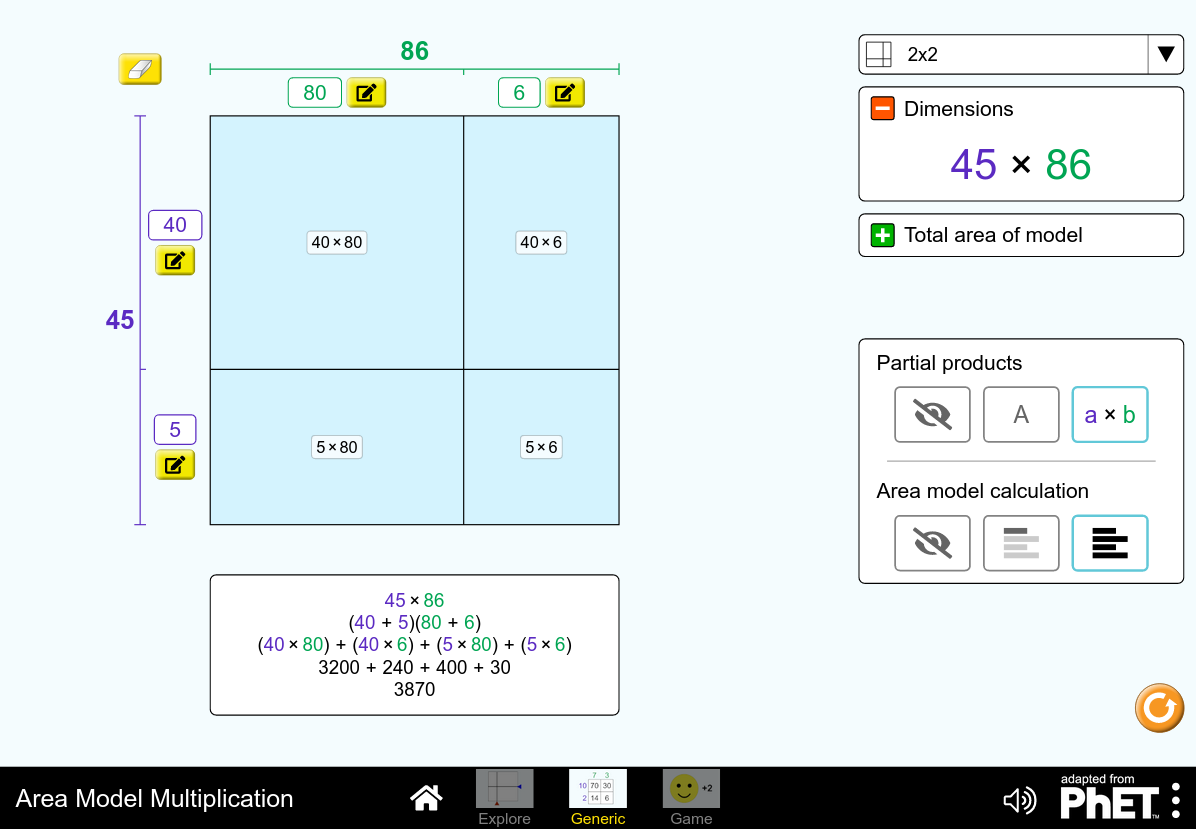MM Practice
×
Multiplication
Division
Place Value
Fractions & Decimals
Measurement
Statistics & Probability
Pre-Algebra
Money
Kindergarten
Geometry

# Multiplying with the Area ModelOnline activity and game for grades 4-5

In this activity, you will see multiplication and partial products illustrated with an area model, and you can practice using this model to multiply numbers (4th and 5th grade math). The sides of the rectangle represent the two numbers to be multiplied, and the area of the rectangle is the product (the answer).

The activity has three parts.

The first part, Explore, lets you drag the sides of the rectangle, and see the corresponding area on the grid. You can choose to reveal the partial products, and the step-by-step calculation of the total area.

The second part, "Generic", allows you to input any numbers to be the side lengths of the rectangle, in two parts. Again, you can reveal the partial products, and the step-by-step calculation of the total area.

The last part is the Game with six levels (of increasing difficulty) where you can really put your skills to practice! You are asked to find the total area, one of the missing side lengths, or one of the partial areas.

Credit: This activity is created by PhET

Screenshots from the game and activity:Allow my comment to be posted on this site